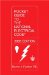# Annex D. Examples

This annex is not part of the requirements of the NFPA document but is included for informational purposes.

Selection of Conductors. In the following examples, the results are generally expressed in amperes (A). To select conductor sizes, refer to the 0 through 2000 Volt (V) ampacity tables of Article 310 and the rules of 310.15 that pertain to these tables.

Voltage. For uniform application of Articles 210, 215, and 220, a nominal voltage of 120, 120/240, 240, and 208Y/120 volts shall be used in calculating the ampere load on the conductor.

Fractions of an Ampere. Except where the calculations result in a major fraction of an ampere (0.5 or larger), such fractions are permitted to be dropped.

Power Factor. Calculations in the following examples are based, for convenience, on the assumption that all loads have the same power factor (PF).

Ranges. For the calculation of the range loads in these examples, Column C of Table 220.55 has been used. For optional methods, see Columns A and B of Table 220.55. Except where the calculations result in a major fraction of a kilowatt (0.5 or larger), such fractions are permitted to be dropped.

SI Units. For metric conversions, 0.093 m2 = 1 ft2 and 0.3048 m = 1 ft.Pocket Guide to the National Electrical Code(R), 2005 Edition (8th Edition)
ISBN: 0131480014
EAN: 2147483647
Year: 2004
Pages: 120